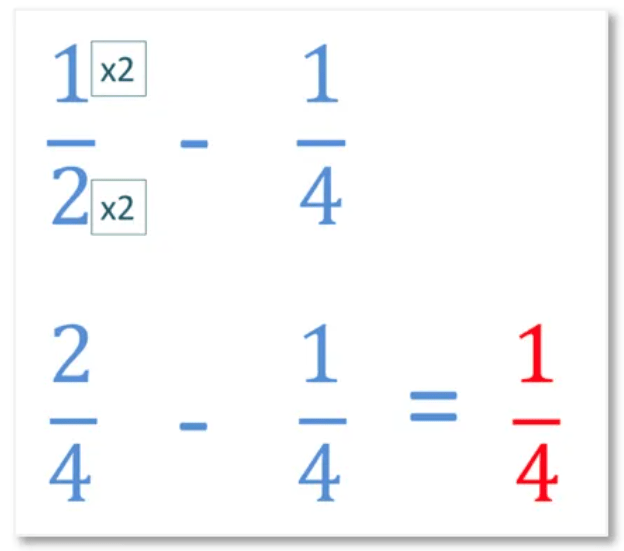# Subtracting Fractions with Like Denominators

Subtracting Fractions with Like Denominators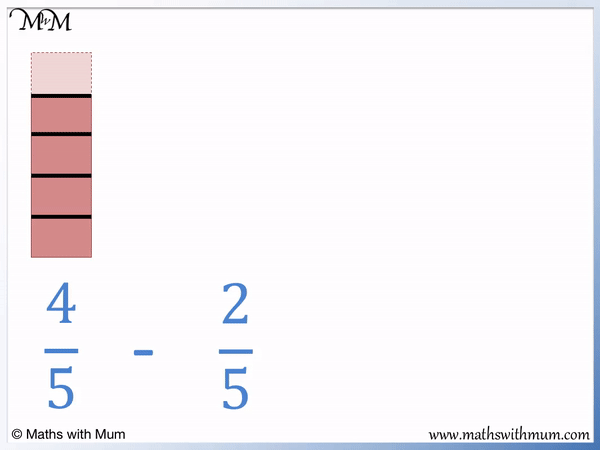• The denominator of a fraction is the number on the bottom of the fraction, below the dividing line.
• Fractions with like denominators are fractions that have the same number on the bottom.
• The numerator is the number on the top of a fraction.
• To subtract fractions with like denominators, we subtract the numerators but keep the denominator the same.
•  4/5   -  2/5   =  2/5  .
• The denominator stays as 5 and we simply work out 4 - 2 = 2 to find the numerator.
To subtract fractions with the same denominators, keep the denominator the same and subtract the numerators.• To subtract fractions with the same denominators, keep the denominator the same and subtract the numerators.
• The denominators are the numbers on the bottoms of the fractions and they are the same in both fractions that we are subtracting.
• The denominators of both fractions are '9' and so the answer will also have a denominator of '9'.
• Now subtract the numerators, which are the numbers on the top of the fractions.
• 8 - 3 = 5.
• 8/9 - 3/9 = 5/9# Subtracting Fractions with Like Denominators

## What are Like Fractions?

Like fractions are fractions that have the same denominator on the bottom of the fraction. For example, the fractions   4/5   and   2/5   are like fractions because they both have a denominator of 5.When adding and subtracting fractions, they must have like denominators. Finding like denominators is an important part of adding and subtracting fractions because the denominator tells us how many parts we have in total. If the number of parts in each fraction is the same, then adding and subtracting the fractions is possible just by looking at the numerators of the fractions.

Like denominators are used to compare two fractions. If fractions have the same denominator, then they can be compared simply by looking at their numerators. The larger the numerator of like fractions, the larger the fraction.

If fractions do not have the same denominator, then equivalent fractions are used to make them like fractions as part of the method to add, subtract or compare them.

## How to Subtract Fractions with Like Denominators

To subtract fractions with like denominators, follow these steps:

1. Keep the denominator of the answer the same as the like denominators.
2. Subtract the numerators to get the numerator of the answer.
3. Simplify the fraction if necessary.

For example,   3/4   -   1/4   =   2/4  .Step 1 is to keep the denominator of the answer the same as the like denominators in the question.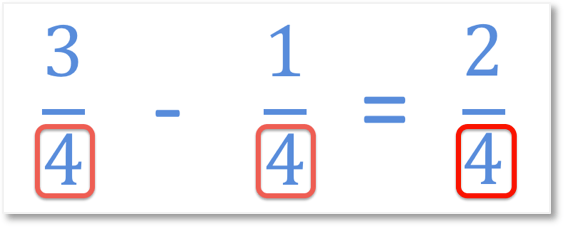Step 2 is to subtract the numerators in the question to find the numerator of the answer.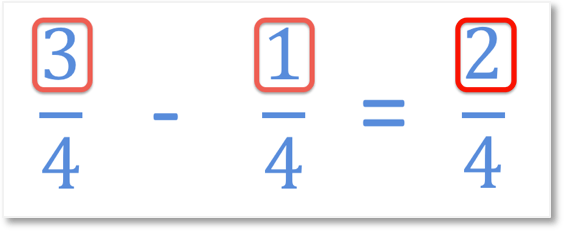3 - 2 = 1 and so, 1 is the numerator of the answer.Step 3 is to simplify the fraction if possible.

2/4   =   1/2  .

To simplify a fraction, divide the numerator and denominator by the same number.

To simplify   2/4   to   1/2  , we divided the numerator and denominator both by 2.

2 is half of 4 and so   2/4   =   1/2  .

Here is another example of subtracting like fractions. We have   8/9   -   3/9   =   5/9  .

Step 1 is to keep the denominator of the answer the same as the denominator of the like fractions in the question.Step 2 is to subtract the numerators. 8 - 3 = 5.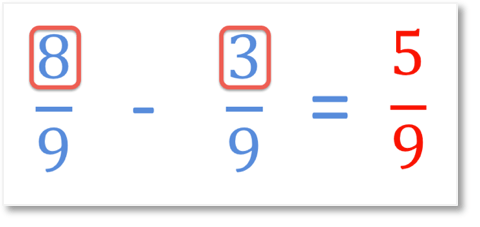8/9   -   3/9   =   5/9  .

Step 3 is to simplify the fraction, however it is not possible to simplify   5/9  . There is no number that divides exactly into both 5 and 9.

Here are some more examples of subtracting fractions with the same denominator.

In this question we are asked to work out   6/6   -   /6  .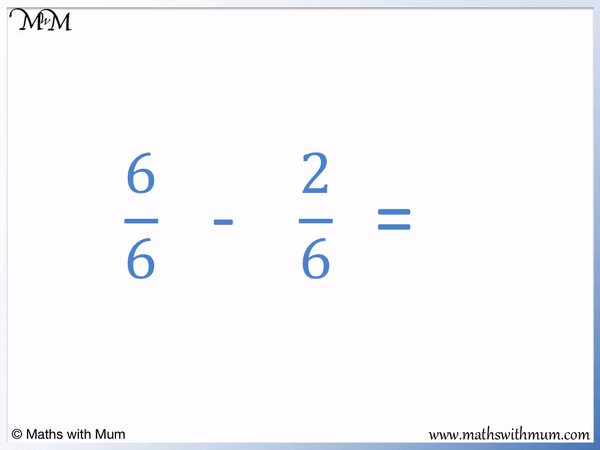The denominator of the fractions is 6 and so, the denominator of the answer is 6.

6 - 2 = 4 and so, the numerator of the answer is 4.

6/6   -   2/6   =   4/6  .

The final step is to simplify the fraction. We can simplify the fraction by halving both the numerator and the denominator since they are both divisible by 2.

4/6   =   2/3  .

In this next example we are asked to work out   7/8   -   3/8  .The first step is to keep the denominator the same. The denominator is 8.

The next step is to subtract the numerators. 7 - 3 = 4, so the numerator is 4.

7/8   -   3/8   =   4/8  .

The fraction   4/8   can be simplified to   1/2   by dividing the numerator and the denominator by 4.

7/8   -   3/8   =   1/2  .

## How to Subtract Improper Fractions with Like Denominators

To subtract improper fractions with like denominators, follow these steps:

1. Keept the denominator of the answer the same as the denominator of the like fractions.
2. Subtract the numerators to get the numerator of the answer.

Subtracting improper fractions uses the same rules as subtracting proper fractions.

An improper fraction is a fraction that has a larger numerator than its denominator.

For example,   13/5   -   6/5   =   7/5  .The first step is to keep the denominator of the answer the same as the denominator of the question. The denominator is still 5.

The next step is to subtract the numerators. 13 - 6 = 7 and so, the numerator of the answer is 7.

## How to Subtract Mixed Numbers with Like Denominators

To subtract mixed numbers with like denominators, follow these steps:

1. Write the fractions as improper fractions.
2. Keep the denominator of the answer the same as the denominator of the like fractions.
3. Subtract the numerators to find the numerator of the answer.
4. Write the fraction as a mixed number again if necessary.

For example, here is 3   1/3   - 1   2/3  .To convert mixed numbers to improper fractions, multiply the whole number part with the denominator and then add the numerator. This gives the numerator of the improper fraction. The denominator of the improper fraction is the same as the denominator of the mixed number.

3 × 3 = 9 and 9 + 1 = 10. Therefore 3   1/3   =   10/3  .

1 × 3 = 3 and 3 + 2 = 5. Therefore 1   2/3   =   5/3  .

We then subtract the improper fractions like usual.

The denominator is kept the same, so the denominator of the answer is still 3.

Subtract the numerators. 10 - 5 = 5 and so, 5 is the numerator of the answer.

5/3   can be written as a mixed number again if necessary.

5 ÷ 3 = 1 remainder 2. The whole number part of the answer is 1 and the remainder of 2 is the numerator of the fraction.

5/3   = 1   2/3  .

3   1/3   - 1   2/3   = 1   2/3  .Now try our lesson on Subtracting Fractions with Unlike Denominators where we learn how to subtract fractions with different denominators.